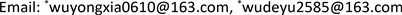﻿ 一类Hamilton矩阵特征值的代数指标 Algebraic Index of Eigenvalue of a Class of Hamilton Matrix

Vol.07 No.03(2018), Article ID:24042,6 pages
10.12677/AAM.2018.73030

Algebraic Index of Eigenvalue of a Class of Hamilton Matrix

Yongxia Wu*, Deyu Wu*, Chunyuan Wang, Ruiting Dong, Yi Shen, Min Xiang

School of Mathematical Science, Inner Mongolia University, Hohhot Inner MongoliaReceived: Feb. 22nd, 2018; accepted: Mar. 7th, 2018; published: Mar. 14th, 2018ABSTRACT

In this paper, the sufficient and necessary conditions of nonnegative Hamilton matrix are proved. Secondly, the problem of when the algebraic index of the eigenvalue of a class of Hamilton matrix is one is studied and the sufficient conditions are given.

Keywords:Hamilton Matrix, Eigenvalues, Eigenvectors, Algebraic Index1. 引言

$H=\left[\begin{array}{cc}A& B\\ C& -{A}^{\ast }\end{array}\right],$

2. 预备知识

${x}^{*}Dx>0\text{\hspace{0.17em}}\left({x}^{*}Dx\ge 0\right),$

${N}^{K}\left(D,\lambda \right)={N}^{K+1}\left(D,\lambda \right),$

${N}^{K}\left(D,\lambda \right)=\left\{u|{\left(D-\lambda I\right)}^{K}u=\text{0}\right\}.$

${\left(D-\lambda I\right)}^{K+1}{u}_{0}=0,\text{}{\left(D-\lambda I\right)}^{K}{u}_{0}\ne 0,$

${\left(D-\lambda I\right)}^{K}{u}_{\text{0}}\in N\left(D,\lambda I\right)$ 。根据给定条件，存在 $v\in {N}^{K}\left({D}^{*},\stackrel{¯}{\lambda }\right)$ 使得

${v}^{\text{*}}{\left(D-\lambda I\right)}^{K}{u}_{0}\ne 0,$

${u}_{0}^{\ast }{\left({D}^{\text{*}}-\stackrel{¯}{\lambda }I\right)}^{K}v\ne 0,$

${x}_{0}^{*}D{x}_{0}={x}_{0}^{*}{P}^{*}P{x}_{0}={\left(P{x}_{0}\right)}^{*}P{x}_{0}=0,$

$P{x}_{0}=0,$

${P}^{*}P{x}_{0}=D{x}_{0}=0.$

3. 主要结果及其证明

$N\left(A\right)\cap N\left(C\right)=\left\{0\right\}$

$N\left({A}^{\text{*}}\right)\cap N\left(B\right)=\left\{0\right\}$

$A{x}_{0}=0,C{x}_{0}=0.$

$u={\left[\begin{array}{cc}{x}_{0}& 0\end{array}\right]}^{\text{T}}$ ，则有

$\left[\begin{array}{cc}A& B\\ C& -{A}^{*}\end{array}\right]\left[\begin{array}{c}{x}_{0}\\ 0\end{array}\right]=\left[\begin{array}{c}A{x}_{0}\\ C{x}_{0}\end{array}\right]=0.$

$N\left(A\right)\cap N\left(C\right)=\left\{0\right\}$$N\left({A}^{\text{*}}\right)\cap N\left(B\right)=\left\{0\right\}.$

$A{x}_{\text{0}}+B{y}_{0}=0,\text{\hspace{0.17em}}\text{\hspace{0.17em}}C{x}_{0}-{A}^{*}{y}_{0}=0.$ (3.1.1)

${x}_{0}^{\ast }C{x}_{\text{0}}+{y}_{0}^{\ast }B{y}_{0}=0,$

${x}_{0}^{\ast }C{x}_{\text{0}}=0,\text{\hspace{0.17em}}\text{\hspace{0.17em}}{y}_{0}^{\ast }B{y}_{0}=0,$

$C{x}_{0}=0,\text{\hspace{0.17em}}\text{\hspace{0.17em}}B{y}_{0}=0,$

$A{x}_{0}=0,\text{\hspace{0.17em}}\text{\hspace{0.17em}}-{A}^{*}{y}_{0}=0.$

$C{x}_{0}=0,\text{\hspace{0.17em}}\text{\hspace{0.17em}}A{x}_{0}=0$

$Ax+By=\lambda x,\text{\hspace{0.17em}}\text{\hspace{0.17em}}Cx-{A}^{*}y=\lambda y,$

${\lambda }^{2}\left({x}^{*}{B}^{-1}x\right)+\lambda \left({x}^{*}{A}^{*}{B}^{-1}x\right)-\lambda \left({x}^{*}{B}^{-1}Ax\right)-\left({x}^{*}Cx\right)-\left({x}^{*}{A}^{*}{B}^{-1}Ax\right)=0.$

$\lambda \left({x}^{*}{A}^{*}{B}^{-1}x\right)-\lambda \left({x}^{*}{B}^{-1}Ax\right)=0,$

$\sigma \left(H\right)\subset R\cup iR$

$\sigma \left(H\right)\subset R$ 时，取 $v=\left[\begin{array}{c}-\lambda {B}^{-1}x-{B}^{-1}Ax\\ -x\end{array}\right]$ ，则

$\left({H}^{*}-\stackrel{¯}{\lambda }\right)v=\left({H}^{*}-\lambda \right)v=J\left(H+\lambda \right)Jv=0,$

$v{}^{*}u=\left(\left[\begin{array}{c}x\\ \lambda {B}^{-1}x-{B}^{-1}Ax\end{array}\right],\left[\begin{array}{c}-\lambda {B}^{-1}x-{B}^{-1}Ax\\ -x\end{array}\right]\right)=-2\lambda \left({x}^{*}{B}^{-1}x\right)\ne 0,$

$\lambda \in iR$ 时，取 $v=\left[\begin{array}{c}-\lambda {B}^{-1}x+{B}^{-1}Ax\\ x\end{array}\right]$ ，则

$\left({H}^{*}-\stackrel{¯}{\lambda }\right)v=\left({H}^{*}+\lambda \right)v=J\left(H-\lambda \right)Jv=0,$

${v}^{*}u=\left(\left[\begin{array}{c}x\\ \lambda {B}^{-1}x-{B}^{-1}Ax\end{array}\right],\left[\begin{array}{c}-\lambda {B}^{-1}x+{B}^{-1}Ax\\ x\end{array}\right]\right)=2\lambda \left({x}^{*}{B}^{-1}x\right)\ne 0,$

${\lambda }_{1}={\lambda }_{2}=0$

${H}^{2}=\left[\begin{array}{cc}0& {I}_{n}\\ 0& 0\end{array}\right]\left[\begin{array}{cc}0& {I}_{n}\\ 0& 0\end{array}\right]=\left[\begin{array}{cc}0& 0\\ 0& 0\end{array}\right]$

$u={\left[\begin{array}{cc}\text{0}& x\end{array}\right]}^{\text{T}}\ne 0$ ，其中 $x$$n$ 维非零向量，则

$Hu={\left[\begin{array}{cc}x& 0\end{array}\right]}^{\text{T}}\ne 0,$

${H}^{\text{2}}u=0.$

$Ax+By=\text{0},\text{\hspace{0.17em}}Cx-{A}^{*}y=\text{0},$

$u={\left[\begin{array}{cc}x& -{B}^{-1}Ax\end{array}\right]}^{\text{T}},$

$Ju=\left[\begin{array}{cc}0& {I}_{n}\\ -{I}_{n}& 0\end{array}\right]\left[\begin{array}{c}x\\ -{B}^{-1}Ax\end{array}\right]={\left[\begin{array}{cc}-{B}^{-1}Ax& -x\end{array}\right]}^{\text{T}}\in N\left({H}^{*}\right),$

${\left(Ju\right)}^{\text{*}}u=\left(\left[\begin{array}{c}x\\ -{B}^{-1}Ax\end{array}\right],\left[\begin{array}{c}-{B}^{-1}Ax\\ -x\end{array}\right]\right)={x}^{*}\left({B}^{-1}A-{A}^{*}{B}^{-1}\right)x\ne 0,$

Algebraic Index of Eigenvalue of a Class of Hamilton Matrix[J]. 应用数学进展, 2018, 07(03): 243-248. https://doi.org/10.12677/AAM.2018.73030

1. 1. 冯康. 哈密尔顿系统的辛几何算法[M]. 杭州: 浙江科技出版社, 2003.

2. 2. 吴德玉, 阿拉坦仓. 非负Hamilton算子的可逆性[J]. 数学年刊A辑(中文版), 2008, 29(5): 719-724.

3. 3. 朱元国, 饶玲, 严涛, 张军, 李宝成. 矩阵分析与计算[M]. 北京: 国防工业出版社, 2010.

4. 4. 吴德玉, 阿拉坦仓. 分块算子矩阵谱理论及其应用[M]. 北京: 科学出版社, 2013.